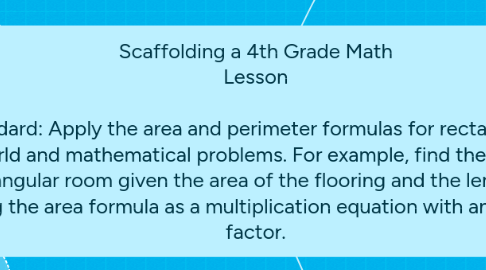# Scaffolding a 4th Grade Math Lesson Standard: Apply the area and perimeter formulas for rectangl...

Get Started. It's FreeScaffolding a 4th Grade Math Lesson Standard: Apply the area and perimeter formulas for rectangles in real-world and mathematical problems. For example, find the width of a rectangular room given the area of the flooring and the length, by viewing the area formula as a multiplication equation with an unknown factor.## 3. Scaffolding Strategies

### 3.1. Show and Tell

3.1.1. I will show students what I am doing rather than just talking about it. As I explain area and perimeter, I will be measuring an object and physically showing them what that looks like, and how to use those measurements to compute area and perimeter.

### 3.2. Think Aloud

3.2.1. Model thinking out loud. While working through a problem, I will say all of my thoughts out loud so that students can have a model for how to think about a problem as they work through it.

### 3.3. Pre-teach Vocabulary

3.3.1. Review definitions of important words for this standard, including: Area, Perimeter, Unit, Centimeters, Inches, Length, Width

### 3.4. Visual Aids

3.4.1. Provide a diagram/picture on a posterboard that shows area and perimeter, and how to find it. Keep these diagrams/visuals up throughout the course of teaching this standard.

### 3.5. Guided Notes

3.5.1. Create and fill out guided notes as a class. This can be an ongoing note sheet that includes concepts taught over the course of several days. The notes will help students to remember the formulas and will also include examples.

### 3.6. Small Groups

3.6.1. Before moving to independent work, students will work in small groups or partners to get comfortable with the material and have a chance to discuss their ideas and thoughts.

### 3.7. I Do, We Do, You Do

3.7.1. I will explain and show studetnts how to do a problem. We will do a problem together. Students will complete a problem on their own.

### 3.8. Prompting

3.8.1. When working one-on-one with a student, i will use prompting. For example, if a student is struggling with an area problem, I can provide them with a prompt by saying, "Okay, so can you tell me what area means?" student answers "Great, so you are saying that area is all the space inside the shape. So how can we figure out how much space is inside this rectangle?"

### 3.9. White Boards

3.9.1. This will be a whole class activity, where students have personal white boards. A problem will be given, and students will complete the problem on their board, then hold up the board to give their answer. Its a quick way for me to check how students are doing and I can provide extra help where needed.